User talk:Jaycall

Joel's First Talk Message to Jay

Jay: In the email message that you sent to me today, you indicated that you had added the 3rd component to the dx/dt equation. I don't see that modification in the current T3Coordinates page. --Tohline 15:05, 29 May 2010 (MDT)

Joel: It's at the bottom of the section entitled "Time-Derivative of Position and Velocity Vectors". Under the history tab, can you see the edits that I made? It was the very first edit and was fairly minor. I only added a couple terms. --Jaycall 20:17, 29 May 2010 (MDT)
The mediaWiki "talk" page recommends that when you reply to a query, you should not start a new subsection but, rather, just indent (using one or more colons) immediately following the query. Also, it recommends that you "sign" each "talk" message. You do this by typing 2 dashes followed by 4 tildes! I'm going to edit your "reply" (and this additional one from me) to put it in this recommended format. But you have to "sign" your own remark. --Tohline 16:23, 29 May 2010 (MDT)
By the way, I can see the edits that you made to add the 3rd component to the dx/dt equation. I did not spot the changes earlier, but via the history "diff" function, I'm able to see the edits clearly. --Tohline 16:41, 29 May 2010 (MDT)

Killing Vector Approach

Thanks for writing out all the terms in the expression for dΞ / dt in your "Killing Vector Approach" discussion. On the one hand, I'm happy that the term I mentioned cancels with another one because that means we have fewer terms to integrate. On the other hand, it was one term that I thought we might actually be able to manipulate analytically. Of the two terms that remain, one is relatively simple — the one that is proportional to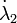$\dot{\lambda}_2$ — but the first term is a bear! That will take some thinking! --Tohline 10:50, 30 May 2010 (MDT)

I know it seems like the wrong direction, but unless we can express this summary integral entirely in terms of λ1, λ2,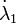$\dot{\lambda}_1$ and$\dot{\lambda}_2$, I think that in order to make progress we're going to have to write things out in terms of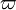$\varpi$, z,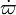$\dot{\varpi}$ and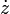$\dot{z}$. Of course, that will make the expression much messier, but I think it will be very difficult to recognize this quantity as an exact derivative unless everything's in terms of the same coordinates. --Jaycall 12:55, 30 May 2010 (MDT)
I agree. It is for similar reasons that I am planning on focusing on the case where q2 = 2. At least in this special case we can invert the coordinate expressions to give$\varpi$ and z in terms of the λs. Incidentally, I have added a link to your "Killing Vector" page as well as a link to this associated "Talk" page on the page where I itemize my various "ramblings". --Tohline 16:16, 30 May 2010 (MDT)
Good point. That would be a good reason to focus on one specific case. I haven't looked closely at the inverted coordinate transformation for the q2 = 2 case for several days. Since the relation is quadratic, is it obvious which root should be used?
The proper physical root was obvious to me when I performed the coordinate inversion in the case of T1 Coordinates, so I presume it will be obvious for the T3 Coordinate system. But the inversion needs to be redone for the case of T3 Coordinates; do you want to take care of this and type up the result? In the (quadratic) case of q2 = 2, it may be as simple as replacing χ2 with 1 / λ2. --Tohline 11:21, 31 May 2010 (MDT)
Sure. --Jaycall 13:34, 31 May 2010 (MDT)

Mistake? Please check the next to last row in the tabulated expressions for Christoffel symbols; shouldn't the i and j indexes be swapped? --Tohline 09:39, 6 June 2010 (MDT)

You are correct. Good eye.--Jaycall 09:42, 9 June 2010 (MDT)
Actually, it wasn't my good eye. I discovered this from my inelegant brute-force derivations. In particular, when I was trying to derive the same form of the equation of motion from two different points of view, I had to plug in some of your Christoffel symbol expressions. At first I could not get my EOM to match yours; it was while trying to understand this mismatch that I realized that your general expression for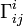$\Gamma^i_{ij}$ did not match the specific expression for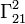$\Gamma^2_{21}$ that you typed on the "T3 Coordinates" page. In my effort to get the EOMs to match, I decided that your general expression was the incorrect one. --Tohline 14:58, 9 June 2010 (MDT)
For the record, on 10 June 2010, I modified the table that contains the general Christoffel symbol expressions in order to correct the order of these indexes.--Tohline 18:55, 11 June 2010 (MDT)

Logarithmic Derivatives of T3 Scale Factors

Check out my new subsection (under T3 Coordinates) entitled Logarithmic Derivatives of Scale Factors. First, see if you agree that there is a mistake (typo) in one of your tables of partial derivatives. Specifically, I think that the correct expression is: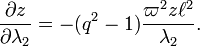$\frac{\partial z}{\partial\lambda_2} = - (q^2-1)\frac{\varpi^2 z \ell^2}{\lambda_2} .$

Second, see if you agree with my derived expressions for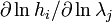$\partial\ln h_i/\partial\ln\lambda_j$. --Tohline 22:28, 31 May 2010 (MDT)

You're certainly right about the sign error. I have corrected the expression in the relevant table. --Jaycall 14:28, 1 June 2010 (MDT)
I have not yet been able to confirm your expressions for logarithmic derivatives of the scale factors. I'm not sure what approach you took in deriving them, but since I had already calculated partials of the scale factors (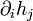$\partial_i h_j$ and so forth), I derived a little trick to help simplify the work. Do you agree that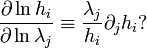$\frac{\partial \ln h_i}{\partial \ln \lambda_j} \equiv \frac{\lambda_j}{h_i} \partial_j h_i ?$

Yes, this is precisely how I defined the logarithmic derivatives. And I used your tables of partial derivatives (e.g.,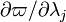$\partial\varpi/\partial\lambda_j$ and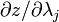$\partial z/\partial\lambda_j$) to obtain$\partial_i h_j$ and so forth. --Tohline 17:30, 1 June 2010 (MDT)

Mistake! Jay: I have found a mistake in my original derivation of the logarithmic derivatives of the h1 scale factor. Perhaps now they will match yours. Check it out and let me know! --Tohline 12:41, 4 June 2010 (MDT)

Joel: I am finally able to confirm your derivation of the logarithmic derivatives of the scale factors. I came at them from a different angle with a fresh head, and I am now confident that what you have is correct. Next I will look into the general case. --Jaycall 14:29, 10 July 2010 (MDT)

Two Views of Equation of Motion

Jay: I have created a new wiki page to explicitly analyze how your Characteristic Vector formalism applies to the T3 Coordinate system. On this page is a subsection entitled "Two Views of Equation of Motion" in which I have shown that the 2nd component of the equation of motion can be written in the following form,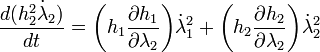$\frac{d(h_2^2 \dot{\lambda}_2)}{dt} = \biggl( h_1 \frac{\partial h_1}{\partial \lambda_2} \biggr) \dot{\lambda}_1^2 + \biggl( h_2 \frac{\partial h_2}{\partial \lambda_2} \biggr) \dot{\lambda}_2^2$ ,

assuming that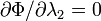$\partial\Phi/\partial\lambda_2 = 0$. More to the point, I have shown that I can derive this form of this component of the equation of motion using either (A) your Christoffel symbol formalism, or (B) the more classical formalism that I have been using, which I obtained from Appendix 1.B of Binney & Tremaine (1987) and Morse & Feshbach (1953).

Now, I shouldn't be shocked that both derivations lead to the same form of the equation — after all, we're doing physics aren't we? But here is what surprised me: The Christoffel symbol formalism produces this form of the equation without ever assuming a particular coordinate system whereas, in order to derive the equation using the more classical formalism, I plugged in some relations between the partial derivatives of the scale factors that I thought were specific to T3 coordinates. This leads me to ask, "How many of the T3 Coordinate relations derived earlier are generic relations that apply to any orthogonal, axisymmetric coordinate system, and how many are unique to the T3 Coordinate System?" I'm particularly interested in knowing how generalizable the relations are that appear inside boxes labeled "T3 Coordinates" under the subsections I have entitled, "Derived Identity for T3 Corodinates" and "Logarithmic Derivatives of Scale Factors". Can you answer this? --Tohline 10:46, 7 June 2010 (MDT)

Joel: Yes, this is the power of using a covariant formulation. The only assumptions we made were that the coordinates were orthogonal and axisymmetric. I believe that the relation under "Derived Identity for T3 Coordinates" would apply to any coordinate system meeting these criteria. I will double check, though. (How did you determine that the position vector could be written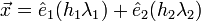$\vec{x} = \hat{e}_1 (h_1 \lambda_1) + \hat{e}_2 (h_2 \lambda_2)$?)
The logarithmic derivatives of the scale factors cannot be general in their current form due to the appearance of factors of q, but I suspect they can be generalized and molded into a form that closely resembles what you have. I will work on this, too. --Jaycall 10:39, 9 June 2010 (MDT)
Jay: You asked how I determined the expression for the position vector. I don't have my detailed notes with me right now, but I can outline the steps. In Morse & Feshbach's classical presentation of orthogonal curvilinear coordinates, they define a set of so-called direction cosines, γij. (I don't have the generalized definition with me at this time, but they are expressible in terms of derivatives of the scale factors with respect to the various coordinates; I'm certain direction cosines are definable in terms of Christoffel symbols.) Each of the Cartesian unit vectors (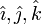$\hat{\imath},\hat{\jmath},\hat{k}$) can be written in terms of products of these direction cosines and the three unit vectors of your chosen curvilinear coordinate system (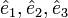$\hat{e}_1, \hat{e}_2, \hat{e}_3$). According to Morse & Feshbach, for example,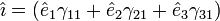$\hat{\imath} = (\hat{e}_1\gamma_{11} + \hat{e}_2\gamma_{21} + \hat{e}_3\gamma_{31})$. So, all I did to obtain an expression for the position vector in T3 coordinates was define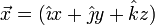$\vec{x} = (\hat\imath x + \hat\jmath y + \hat{k}z)$, then replace each Cartesian unit vector by its expression in terms of products of the T3-coordinate unit vectors & the appropriate direct cosine, then gather together and simplify terms. Does this make sense? --Tohline 14:59, 10 June 2010 (MDT)
Yes, it does. That's very helpful. --Jaycall 09:49, 11 June 2010 (MDT)
Jay: For the record, I have put together a wiki page that discusses the definition and utility of Direction Cosines.--Tohline 18:47, 3 July 2010 (MDT)

Joel, I finally figured out what was going on this afternoon with the strange conserved quantities we were getting, like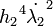${h_2}^4 \dot{\lambda_2}^2$ and, consequently, λ1λ2. There is a mistake in the derivation stemming all the way back from Eq. CV.02 on my page on the characteristic vector approach. My derivation of the brute force condition on C2 was correct, but you can see from above that Ξ does not equal lnC2, it equals − lnC2. Consequently, the conclusion is no longer that C2 must equal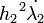${h_2}^2 \dot{\lambda_2}$, but rather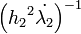$\left( {h_2}^2 \dot{\lambda_2} \right)^{-1}$. So in fact you did find a conserved quantity--1!  : ) I guess it was a tautology after all; your intuition was right. --Jaycall 18:50, 14 July 2010 (MDT)

Jay, very good catch! Looking back through the history of that wiki page, it is clear that I am the one who introduced this sign error. I mistakingly set Ξ = lnC2 in equation CV.02 at the same time that I "Added a couple of right-justified equation numbers." I'll march through the relevent wiki pages today and attempt to correct this mistake wherever it appears. --Tohline 08:30, 15 July 2010 (MDT)
Joel, I'm sorry yesterday's excitement didn't pan out. It would have been really neat. Well, at least I learned something. On my drive home yesterday I was thinking about what it meant for there to be a constraint that involved only the coordinates (and not their rates of change). We were uncomfortable with this idea, but now I understand why in general no conserved quantity can take this form. If there were a constraint on only the coordinates, then this would limit a particle to a curve or a surface...a subspace of physical space (as opposed to a subspace of phase space). Yet it would definitely be possible to get a particle to leave the subspace by giving it an initial velocity in any direction normal to the subspace. Any conserved quantity must definitely involve the coordinate velocities (unless you're artificially restricting the motion). --Jaycall 14:15, 15 July 2010 (MDT)
Good observation. --Tohline 16:28, 15 July 2010 (MDT)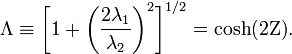$\Lambda \equiv \biggl[1 + \biggl(\frac{2\lambda_1}{\lambda_2}\biggr)^2\biggr]^{1/2} = \cosh(2\Zeta) .$
By the way, if you don't agree that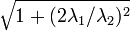$\sqrt{1+(2\lambda_1/\lambda_2)^2}$ equals cosh(2Ζ), please let me know!--Tohline 19:49, 11 June 2010 (MDT)